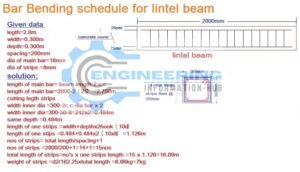# How To Find The Bar Bending Schedule for Lintel Beam

## How To Find The Bar Bending Schedule for Lintel Beam | BBS For Lintel Beam

### what is the lintel beam?

The lintel beam is the type of horizontal beam. this beam is put on the above door or windows. the lintel beam is the main purpose to support the load.How To Find The Bar Bending Schedule for Lintel Beam

in this article, we calculate the bar bending schedule for the lintel beam. bar bending schedule means the calculate the rebar using in lintel beam.

we have some dimensions of the lintel beam to use for help to calculate the rebar or make a bar bending schedule.

###length of lintel beam =2800mm=2.8m

width of lintel beam =300mm=0.300m

depth of lintel beam =300mm=0.300m

dia of the main bar = 16mm

no’s of main bar = 4no’s

dia of strips =8mm

spacing of strips =200mm

concrete cover=25mm

now we calculate the rebar

# Bar Bending Schedule of Circular Column

length of the main bar = total length of lintel beam-2.concrete cover

length of the main bar =2800-2(25)

length of the main bar =2800-50=2750=2.750m

no’s of main bar = 4

the total length of the main bar = no’s of bar ×length of one bar

the total length of the main bar =4×2.750=11m

weight of the main bar =d²/162.25×total length

weight of the main bar =16²/162.25×11=17.35kg=18kg

cutting length of strips

dia of strips inner depth side =width-2concrete cover-dia of bar×2

dia of strips inner depth side=300-2(25)-8×2

dia of strips inner depth side=300-50-8×2

dia of strips inner depth side=300-58=242×2=484mm=0.484m

dia of strips inner width side is same= 0.484m

cutting length of strips =dia of width+dia of depth+2hooks(10d)

cutting length of strips =0.484+0.484+2(10×8)

cutting length of strips =0.484+0.484+0.160=1.128m

no’s of stips =total length of lintel beam/spacing+1

no’s of stips =2800/200+1=14+1=15no’s

the total length of strips =no’s of strips × length of one strip

the total length of strips =15×1.128=16.92m

weight of strips =d²/162.5×total length of strips

weight of strips =8²/162.25×16.92=6.67kg=7kg